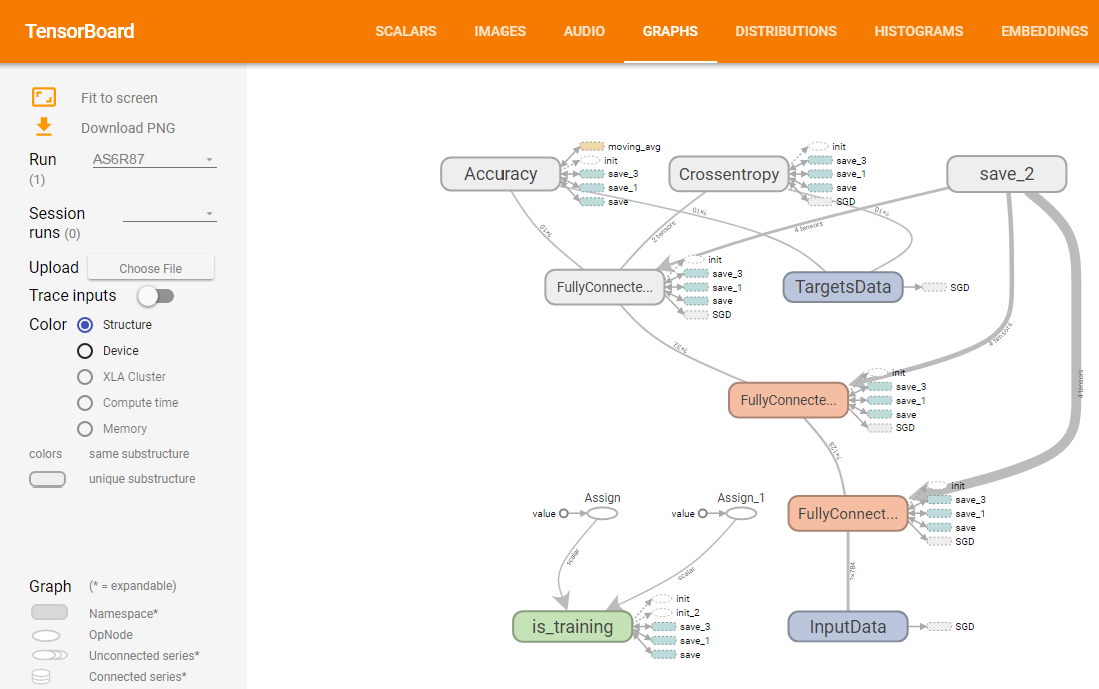# Tech Tips

1. Uncategorized
2. 1397 view

## Visualization of Neural Network and its Training using TensorboardContents

# Introduction

Sometimes I guess you think you want to visualize Neural Networks and see learning curve immediately when you use tensorflow and tflearn. I found a good tool. It’s called tensorboard. I’ll show you how to use it. You can try samples easily in this article with following Docker image.

# Environment

I used tflearn to make model easily.
• OS : Ubuntu 16.04
• python : 3.5.2
• tensorflow : 1.1.0
• tfLearn : 0.3
• tensorboard : 1.0.0a6

# Install tensorboard

It’s super easy. Only pip install command is only you need to run. But, looks I couldn’t run tensorboard in virtualenv. So, if you meet a problem with virtualenv, please try it out of virtualenv. If you haven’t prepared python env, this article will help you.
```pip install tensorboard
```

# Sample Neural Network

I’ll create a model to recognize hand-written digits using MNIST dataset. If you don’t have numpy, tensorflow and tflearn, please install with pip install command. I’ll create a very simple model. Number of input layer units is 784(28 x 28 pixel). There are 2 hidden layers. One of them is 128 units and the other’s number of units is 32. Number of output layer’s unit is 10 to output digits from 0 to 9. Actual code is following.
```import numpy as np
import tensorflow as tf
import tflearn
import tflearn.datasets.mnist as mnist

X_train, y_train, X_test, y_test = mnist.load_data(one_hot=True)

# 2. Build a NN Model
tf.reset_default_graph()
net = tflearn.input_data([None, X_train.shape]) # Input Layer
net = tflearn.fully_connected(net, 128, activation='ReLU') # Hidden Layer 1
net = tflearn.fully_connected(net, 32, activation='ReLU') # Hidden Layer 2
net = tflearn.fully_connected(net, 10, activation='softmax') # Output Layer
net = tflearn.regression(net, optimizer='sgd', learning_rate=0.01, loss='categorical_crossentropy')
model = tflearn.DNN(net, tensorboard_verbose=3)

# 3. Traning
model.fit(X_train, y_train, validation_set=0.1, show_metric=True, batch_size=100, n_epoch=20)

# 4. Testing
predictions = np.array(model.predict(X_test)).argmax(axis=1)
actual = y_test.argmax(axis=1)
test_accuracy = np.mean(predictions == actual, axis=0)
print("Test accuracy: ", test_accuracy)
```
Result of my trial is around 91% accuracy.

# See The Model and Traning Status on Tensorboard

tflearn automatically outputs log files into /tmp/tflearn_logs. Tensorboard can use the log file. Tensorboard is WebUI and it’s default port is 6006. You can launch it with following command.
```tensorboard --logdir='/tmp/tflearn_logs' --port=6006
```
You can see the results with accessing to like http://localhost:6006 by browser. GRAPHS tab shows model topology and training information. SCALARS tab shows training status. If you train the model sometimes, you can see multiple results on the tab.

# Take a Look at a Model on Jupyter Notebook

If you want to take a look at a Neural Network model which you created on Jupyter Notebook, you can use tfgraphviz. You can install tfgraphviza with just running pip install.
```pip install tfgraphviz
```
I couldn’t output a model which I created using tflearn. So, in this time I used tensorflow. I used this article’s sample .
```import numpy as np
import tensorflow as tf

tf.reset_default_graph()

# Creating input and correct result data
x_data = np.random.rand(100).astype(np.float32)
y_data = x_data * 0.1 + 0.3

# Build network
W = tf.Variable(tf.random_uniform(, -1.0, 1.0))
b = tf.Variable(tf.zeros())
y = W * x_data + b
loss = tf.reduce_mean(tf.square(y - y_data))
train = optimizer.minimize(loss)

# Output graph with tfgraphviz
tfg.board(tf.get_default_graph())
```
The result is following. This is not very easy to understand at first look. But, you can understand overview.

# Docker image

You can try samples in this article immediately using following image. You can run Jupyter notebook and Tensorboard by following commands. The image has samples.ipynb which has this article’s all codes.
```### Docker Pull
docker pull zuqqhi2/ml-python-sandbox:latest
docker images
#REPOSITORY                  TAG                 IMAGE ID            CREATED             SIZE
#zuqqhi2/ml-python-sandbox   latest              4402825ff756        2 hours ago         12.9 GB

### Run jupyter without login to container
docker run -it -p 8888:8888 -p 6006:6006 zuqqhi2/ml-python-sandbox
```

### Comment

1. For Alex, your comment is saved at JP version article page.
So, let me put your comment and my answer for you here.

****************************************************************
Alex Nordeen

Hi,

I’ve reached out several times but haven’t heard back, which tells me one of three things:
1. You’re interested in giving link back, but haven’t had a chance to get back to me yet.
2. You’re not interested and want me to stop emailing.
3. You’ve fallen and can’t get up – in that case let me know and I’ll call 911.
Can you please reply with 1, 2 or 3? I don’t want to be a bother.

======================Original Message========================

Hi,

Saw your post on https://zuqqhi2.com/en/visualization-neural-network-training-using-tensorboard, and noticed that you’ve shared http://tflearn.org/.

Just thought that this piece on TENSOR I recently published might be valuable to your readers/followers as well.

https://www.guru99.com/tensorflow-tutorial.html

Cheers!
Alex

****************************************************************

Hi Alex,

Thank you for giving messages to me.
And I’m very sorry.
Just I was suffered from spams and I couldn’t find your comment.

Thank you for sharing your site.
I just took a look at but looks very useful!